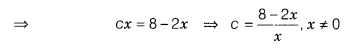# For what value of c in terms of x, the linear equation 2x + cy = 8 has equal values of x,y

For what value of c in terms of x, the linear equation 2x + cy = 8 has equal values of x and y for its solution?

Given linear equation is
2x + cy = 8 …(i)
Now, by given condition, xand y-coordinates of given linear equation are same, i.e. x = y.
On putting y = x in Eq. (i), we get
2x + cx = 8which is the required value of c.